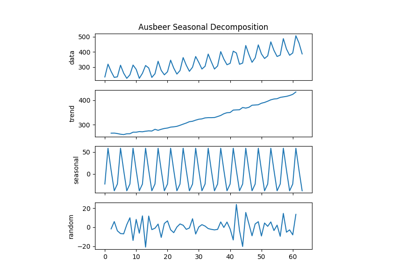# pmdarima.utils.decomposed_plot¶

pmdarima.utils.decomposed_plot(decomposed_tuple, figure_kwargs=None, show=True)[source][source]

Plot the decomposition of a time series.

Plots the results of the time series decomposition in four plots: the ‘x’, ‘trend’, ‘seasonal’, and ‘random’ components.

Parameters: decomposed_tuple : tuple, namedtuple or iterable Named tuple of series that consist of data, trend, seasonal, and random. Should be the result of pmdarima.arima.decompose(). figure_kwargs : dict, optional (default=None) Optional dictionary of keyword arguments that are passed to figure. show : bool, optional (default=True) Whether to show the plot after it’s been created. If not, will return the plot as an Axis object instead.

Notes

This method will only show the plot if show=True (which is the default behavior). To simply get the axis back (say, to add to another canvas), use show=False.

## Examples using pmdarima.utils.decomposed_plot¶